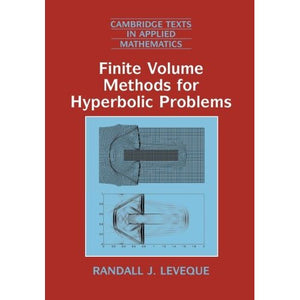># Finite Volume Methods for Hyperbolic Problems: 31 (Cambridge Texts in Applied Mathematics, Series Number 31)

• £36.99
• Save £23

Randall J. Leveque
Cambridge University Press, 8/29/2002
EAN 9780521009249, ISBN10: 0521009243

Paperback, 578 pages, 24.6 x 17.5 x 2.8 cm
Language: English

This book, first published in 2002, contains an introduction to hyperbolic partial differential equations and a powerful class of numerical methods for approximating their solution, including both linear problems and nonlinear conservation laws. These equations describe a wide range of wave propagation and transport phenomena arising in nearly every scientific and engineering discipline. Several applications are described in a self-contained manner, along with much of the mathematical theory of hyperbolic problems. High-resolution versions of Godunov's method are developed, in which Riemann problems are solved to determine the local wave structure and limiters are then applied to eliminate numerical oscillations. These methods were originally designed to capture shock waves accurately, but are also useful tools for studying linear wave-propagation problems, particularly in heterogenous material. The methods studied are implemented in the CLAWPACK software package and source code for all the examples presented can be found on the web, along with animations of many of the simulations. This provides an excellent learning environment for understanding wave propagation phenomena and finite volume methods.

Preface
1. Introduction
2. Conservation laws and differential equations
3. Characteristics and Riemann problems for linear hyperbolic equations
4. Finite-volume methods
5. Introduction to the CLAWPACK software
6. High resolution methods
7. Boundary conditions and ghost cells
8. Convergence, accuracy, and stability
9. Variable-coefficient linear equations
10. Other approaches to high resolution
11. Nonlinear scalar conservation laws
12. Finite-volume methods for nonlinear scalar conservation laws
13. Nonlinear systems of conservation laws
14. Gas dynamics and the Euler equations
15. Finite-volume methods for nonlinear systems
16. Some nonclassical hyperbolic problems
17. Source terms and balance laws
18. Multidimensional hyperbolic problems
19. Multidimensional numerical methods
20. Multidimensional scalar equations
21. Multidimensional systems
22. Elastic waves
23. Finite-volume methods on quadrilateral grids
Bibliography
Index.

'It is moreover advantageous that the algorithms presented are public and easily available via the web-page cited. Similar to the book of R. J. LeVeque titled Numerical Methods for Conservation Laws, this manuscript will certainly become a part of the standard literature in the field of numerical methods for hyperbolic partial differential equations.' Journal of Applied Mathematics and Physics